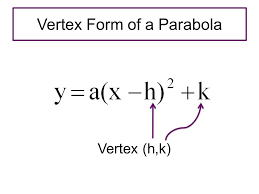# Standard Form of a Quadratic Function

y=x2+x+

### Solution with Steps

Enter the 3 coefficients a,b,c of the Quadratic Equation in the above 3 boxes.
Next, press the button to find the Vertex and Vertex Form with Steps.

### What you need to know about Standard Form of a Quadratic Function

The Quadratic Equation in Standard Form is
\
 ax²+bx+c = 0 }
Then, the Vertex (h,k) can be found from the above Standard Form using
\  h= {-b \over 2a} , k=f( {-b \over 2a })  }

Once computed, the vertex coordinates are plugged into the Vertex Form of a Parabola, see below.Locating the Vertex on the Graph of any Parabola
Every Parabola has either a minimum (when opened to the top) or a maximum (when opened to the bottom).
The Vertex is just that particular point on the Graph of a Parabola.
See the illustration of the two possible vertex locations below:Sample Problem: How to convert the Standard Form of a Quadratic Function to Vertex Form

We are given the Standard Form y=3x²- 6x-2 . First, compute the x-coordinate of the vertex  h={ - b \over 2a}= { -(-6)\over (2*3)} = 1 .Next, compute the y-coordinate of the vertex  by plugging -1 into the given equation: k= 3*(1)²-6(1)-2=-5 .

Therefore, the vertex is (h,k)=(1,-5) .

Thus, we transformed the above Standard Form into the Vertex Form   y=(x-1)²-5  . Easy, wasn't it?

Tip: When using the above Standard Form Calculator to solve   3x²-6x-2=0 we must enter the 3 coefficients as a=3, b=-6 and c=-2.

Then, the calculator will find the Vertex (h,k)=(1,-5)  step by step.

Finally, the vertex form of the Parabola is   y=(x-1)²-5   . Get it now? Try the above Standard Form Calculator again.